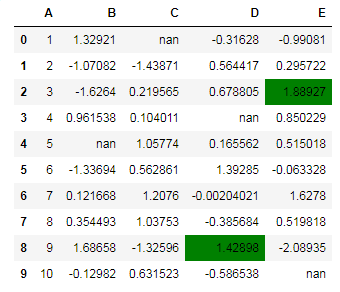﻿ Pandas styling: Write a Pandas program to highlight the maximum value in last two columns - w3resource# Pandas styling Exercises: Write a Pandas program to highlight the maximum value in last two columns

## Pandas styling: Exercise-5 with Solution

Create a dataframe of ten rows, four columns with random values. Write a Pandas program to highlight the maximum value in last two columns.

Sample Solution :

Python Code :

``````import pandas as pd
import numpy as np
np.random.seed(24)
df = pd.DataFrame({'A': np.linspace(1, 10, 10)})
df = pd.concat([df, pd.DataFrame(np.random.randn(10, 4), columns=list('BCDE'))],
axis=1)
df.iloc[0, 2] = np.nan
df.iloc[3, 3] = np.nan
df.iloc[4, 1] = np.nan
df.iloc[9, 4] = np.nan
print("Original array:")
print(df)
def highlight_max(s):
'''
highlight the maximum in a Series green.
'''
is_max = s == s.max()
return ['background-color: green' if v else '' for v in is_max]

print("\nHighlight the maximum value in last two columns:")
df.style.apply(highlight_max,subset=pd.IndexSlice[:, ['D', 'E']])
``````

Original array:

```Original array:
A         B         C         D         E
0   1.0  1.329212       NaN -0.316280 -0.990810
1   2.0 -1.070816 -1.438713  0.564417  0.295722
2   3.0 -1.626404  0.219565  0.678805  1.889273
3   4.0  0.961538  0.104011       NaN  0.850229
4   5.0       NaN  1.057737  0.165562  0.515018
5   6.0 -1.336936  0.562861  1.392855 -0.063328
6   7.0  0.121668  1.207603 -0.002040  1.627796
7   8.0  0.354493  1.037528 -0.385684  0.519818
8   9.0  1.686583 -1.325963  1.428984 -2.089354
9  10.0 -0.129820  0.631523 -0.586538       NaN

Highlight the maximum value in last two columns:
```

Sample Output:

``````

Python Code Editor:

Have another way to solve this solution? Contribute your code (and comments) through Disqus.

What is the difficulty level of this exercise?

﻿

## Python: Tips of the Day

Python: How do I copy a file in Python?

shutil has many methods you can use. One of which is:

```from shutil import copyfile
copyfile(src, dst)
```
• Copy the contents of the file named src to a file named dst.
• The destination location must be writable; otherwise, an IOError exception will be raised.
• If dst already exists, it will be replaced.
• Special files such as character or block devices and pipes cannot be copied with this function.
• With copy, src and dst are path names given as strings.

If you use os.path operations, use copy rather than copyfile. copyfile will only accept strings.

Ref: https://bit.ly/3e2Y1G0